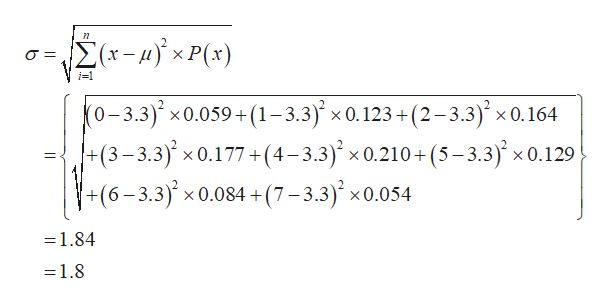# I don't understand how to use the given information to answer the questions about the mean and standard deviation. Can you explain?

Question
1 views

I don't understand how to use the given information to answer the questions about the mean and standard deviation. Can you explain?

check_circle

Step 1

Given probability distribution is:

 Activities 0 1 2 3 4 5 6 7 Probability 0.059 0.123 0.164 0.177 0.21 0.129 0.084 0.054

Explanation:

Let x is random variable.

The mean is calculated as follows:

Step 2

Hence, the mean is 3.3.

The standard devia...help_outlineImage Transcriptionclose-4xP(s) X i-1 (0-3.3)' x0.059+(1-3.3) x 0.123+ (2-3.3) x 0.164 |+(3-3.3)x 0.177+(4-3.3) x 0.210+(5-3.3)x0.129 +(6-3.3) x 0.084 +(7-3.3) x0.054 _ = 1.84 = 1.8 fullscreen

### Want to see the full answer?

See Solution

#### Want to see this answer and more?

Solutions are written by subject experts who are available 24/7. Questions are typically answered within 1 hour.*

See Solution
*Response times may vary by subject and question.
Tagged in

### Measures